﻿ 基于均差估计二变量Meta-分析的随机效应模型 The Random-Effect Model of Bivariate Meta-Analysis Based on the Difference Mean

Pure Mathematics
Vol. 09  No. 01 ( 2019 ), Article ID: 28662 , 13 pages
10.12677/PM.2019.91013

The Random-Effect Model of Bivariate Meta-Analysis Based on the Difference Mean

Pengfei Guo1,2,3, Shuangyin Liu2,3,4, Gang Li5*, Lei Zhang2,3,4,, Liang Cao2,3,4, Xiangru Sun6, Longqin Xu2,3,4

1College of Computational Science, Zhongkai University of Agriculture and Engineering, Guangzhou Guangdong

2Intelligent Agriculture Engineering Research Center of Guangdong Higher Education Institutes, Zhongkai University of Agriculture and Engineering, Guangzhou Guangdong

3Guangdong Province Key Laboratory of Waterfowl Healthy Breeding, Zhongkai University of Agriculture and Engineering, Guangzhou Guangdong

4College of Information Science and Technology, Zhongkai University of Agriculture and Engineering, Guangzhou Guangdong

5Institute of Reproductive Medicine, Panyu Hexian Memorial Hospital of Guangzhou, Guangzhou Guangdong

6Guangzhou E-Government Center, Guangzhou Guangdong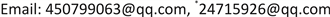Received: Jan. 2nd, 2019; accepted: Jan. 21st, 2019; published: Jan. 28th, 2019ABSTRACT

In this paper, we establish the random-effect model of bivariate meta-analysis based on the difference mean. Under the assumption of the multilevel normal distribution of the difference mean, we obtain the maximum likelihood estimator ${\stackrel{^}{\mu }}_{ML}$ of the difference mean by the method of maximum likelihood estimate. We conclude that the maximum likelihood estimator ${\stackrel{^}{\mu }}_{ML}$ of the difference mean is unbiased for the difference mean effect $\mu$ . In addition, we get the corresponding covariance matrix and the $\left(1-\alpha \right)100%$ confidence interval for the difference mean effect $\mu$ .

Keywords:Meta-Analysis, Maximum Likelihood Estimate, Random-Effect Model1仲恺农业工程学院，计算科学学院，广东 广州

2仲恺农业工程学院，广东省高校智慧农业工程研究中心，广东 广州

3仲恺农业工程学院，广东省水禽健康养殖重点实验室，广东 广州

4仲恺农业工程学院，信息科学与技术学院，广东 广州

5广州市电子政务服务中心，广东 广州

6番禺何贤纪念医院，生殖医学科，广东 广州1. 前言

Meta-分析是一种对同一问题的不同研究进行定量合并的一种方法。该方法是通过考虑每个研究内和研究间的差异性以及研究发表偏倚性得到一个综述结果，从而解决了单个研究样本量小，区域、种族差异大等的缺点。因此Meta-分析的结果对很多领域的科学研究都具有指导意义。针对具体问题的Meta-分析及其统计方法研究已持续了一个世纪之久。最早是在1904年Karl Pearson针对伤寒疫苗效果进行了合并研究  ；接下来的很多年中对某些零星的问题做了类似的综合  。但是在过去的四十年中，人们逐渐意识到医学实验和临床操作需要基于整体的相关性和可靠证据，Meta-分析的影响得到极大的扩展  。仅在2018年，关于健康管理的Meta-分析发表文章将近4000篇。

2. 问题描述

${D}_{i}=\left(\begin{array}{c}{D}_{i1}\\ {D}_{i2}\end{array}\right)\sim N\left(\left(\begin{array}{c}{\mu }_{1}\\ {\mu }_{2}\end{array}\right),\left(\begin{array}{cc}{\sigma }_{i1}^{2}{w}_{i1}+{\tau }^{2}& {\rho }_{0}{\sigma }_{i1}{\sigma }_{i2}\sqrt{{w}_{i1}{w}_{i2}}+{\rho }_{1}\tau \gamma \\ {\rho }_{0}{\sigma }_{i1}{\sigma }_{i2}\sqrt{{w}_{i1}{w}_{i2}}+{\rho }_{1}\tau \gamma & {\sigma }_{i2}^{2}{w}_{i2}+{\gamma }^{2}\end{array}\right)\right)$

3. 均值效应量的极大似然估计

$L\left(\mu ,{D}_{i},{S}_{i}\right)=\underset{i=1}{\overset{k}{\prod }}\frac{1}{2\text{π}det{\left({S}_{i}+\Sigma \right)}^{\frac{1}{2}}}\mathrm{exp}\left\{-\frac{{\left({D}_{i}-\mu \right)}^{\text{T}}{\left({S}_{i}+\Sigma \right)}^{-1}\left({D}_{i}-\mu \right)}{2}\right\}$

$\mathrm{ln}L\left(\mu ,{D}_{i},{S}_{i}\right)=-k\mathrm{ln}\left(2\text{π}\right)-\frac{1}{2}\underset{i=1}{\overset{k}{\sum }}\mathrm{ln}\left[det\left({S}_{i}+\Sigma \right)\right]-\frac{1}{2}\underset{i=1}{\overset{k}{\sum }}{\left({D}_{i}-\mu \right)}^{\text{T}}{\left({S}_{i}+\Sigma \right)}^{-1}\left({D}_{i}-\mu \right)$$L\left(\mu ,{D}_{i},{S}_{i}\right)=\underset{i=1}{\overset{k}{\prod }}\frac{1}{2\text{π}det{\left({S}_{i}+\Sigma \right)}^{\frac{1}{2}}}\mathrm{exp}\left\{-\frac{{\left({D}_{i}-\mu \right)}^{\text{T}}{\left({S}_{i}+\Sigma \right)}^{-1}\left({D}_{i}-\mu \right)}{2}\right\}$

$\begin{array}{c}\mathrm{ln}L\left(\mu ,{D}_{i},{S}_{i}\right)=-k\mathrm{ln}\left(2\text{π}\right)-\frac{1}{2}\underset{i=1}{\overset{k}{\sum }}\mathrm{ln}\left[det\left({S}_{i}+\Sigma \right)\right]-\frac{1}{2}\underset{i=1}{\overset{k}{\sum }}{\left({D}_{i}-\mu \right)}^{\text{T}}{\left({S}_{i}+\Sigma \right)}^{-1}\left({D}_{i}-\mu \right)\\ =-\frac{1}{2}\underset{i=1}{\overset{k}{\sum }}\mathrm{ln}\left[\left({\sigma }_{i1}^{2}{\omega }_{i1}+{\tau }^{2}\right)\left({\sigma }_{i2}^{2}{\omega }_{i2}+{\gamma }^{2}\right)-{\left({\rho }_{0}{\sigma }_{i1}{\sigma }_{i2}\sqrt{{\omega }_{i1}{\omega }_{i2}}+{\rho }_{1}\tau \gamma \right)}^{2}\right]\\ \text{\hspace{0.17em}}\text{\hspace{0.17em}}-\frac{1}{2}\underset{i=1}{\overset{k}{\sum }}{\left[\left({\sigma }_{i1}^{2}{\omega }_{i1}+{\tau }^{2}\right)\left({\sigma }_{i2}^{2}{\omega }_{i2}+{\gamma }^{2}\right)-{\left({\rho }_{0}{\sigma }_{i1}{\sigma }_{i2}\sqrt{{\omega }_{i1}{\omega }_{i2}}+{\rho }_{1}\tau \gamma \right)}^{2}\right]}^{-1}\\ \text{\hspace{0.17em}}\text{\hspace{0.17em}}×\left[\left({\sigma }_{i2}^{2}{\omega }_{i2}+{\gamma }^{2}\right){\left({D}_{i1}-{\mu }_{1}\right)}^{2}-2\left({\rho }_{0}{\sigma }_{i1}{\sigma }_{i2}\sqrt{{\omega }_{i1}{\omega }_{i2}}+{\rho }_{1}\tau \gamma \right)\\ \text{\hspace{0.17em}}\text{\hspace{0.17em}}×\left({D}_{i1}-{\mu }_{1}\right)\left({D}_{i2}-{\mu }_{2}\right)+\left({\sigma }_{i1}^{2}{\omega }_{i1}+{\tau }^{2}\right){\left({D}_{i2}-{\mu }_{2}\right)}^{2}\right]-k\mathrm{ln}\left(2\pi \right)\end{array}$

$\begin{array}{c}\frac{\partial \mathrm{ln}L\left(\mu ,{D}_{i},{S}_{i}\right)}{\partial {\mu }_{1}}=\underset{j=1}{\overset{k}{\sum }}{\left[\left({\sigma }_{i1}^{2}{\omega }_{i1}+{\tau }^{2}\right)\left({\sigma }_{i2}^{2}{\omega }_{i2}+{\gamma }^{2}\right)-{\left({\rho }_{0}{\sigma }_{i1}{\sigma }_{i2}\sqrt{{\omega }_{i1}{\omega }_{i2}}+{\rho }_{1}\tau \gamma \right)}^{2}\right]}^{-1}\\ \text{\hspace{0.17em}}\text{\hspace{0.17em}}×\left[\left({\sigma }_{i2}^{2}{\omega }_{i2}+{\gamma }^{2}\right)\left({D}_{i1}-{\mu }_{1}\right)-\left({\rho }_{0}{\sigma }_{i1}{\sigma }_{i2}\sqrt{{\omega }_{i1}{\omega }_{i2}}+{\rho }_{1}\tau \gamma \right)\left({D}_{i2}-{\mu }_{2}\right)\right]\end{array}$

$\begin{array}{c}\frac{\partial \mathrm{ln}L\left(\mu ,{D}_{i},{S}_{i}\right)}{\partial {\mu }_{2}}=\underset{j=1}{\overset{k}{\sum }}{\left[\left({\sigma }_{i1}^{2}{\omega }_{i1}+{\tau }^{2}\right)\left({\sigma }_{i2}^{2}{\omega }_{i2}+{\gamma }^{2}\right)-{\left({\rho }_{0}{\sigma }_{i1}{\sigma }_{i2}\sqrt{{\omega }_{i1}{\omega }_{i2}}+{\rho }_{1}\tau \gamma \right)}^{2}\right]}^{-1}\\ \text{\hspace{0.17em}}\text{\hspace{0.17em}}×\left[-\left({\rho }_{0}{\sigma }_{i1}{\sigma }_{i2}\sqrt{{\omega }_{i1}{\omega }_{i2}}+{\rho }_{1}\tau \gamma \right)\left({D}_{i1}-{\mu }_{1}\right)+\left({\sigma }_{i1}^{2}{\omega }_{i1}+{\tau }^{2}\right)\left({D}_{i2}-{\mu }_{2}\right)\right]\end{array}$

$\frac{\partial \mathrm{ln}L\left(\mu ,{D}_{i},{S}_{i}\right)}{\partial {\mu }_{1}}=0,\frac{\partial \mathrm{ln}L\left(\mu ,{D}_{i},{S}_{i}\right)}{\partial {\mu }_{2}}=0$ 即得：

$\begin{array}{l}\underset{j=1}{\overset{k}{\sum }}{\left[\left({\sigma }_{i1}^{2}{\omega }_{i1}+{\tau }^{2}\right)\left({\sigma }_{i2}^{2}{\omega }_{i2}+{\gamma }^{2}\right)-{\left({\rho }_{0}{\sigma }_{i1}{\sigma }_{i2}\sqrt{{\omega }_{i1}{\omega }_{i2}}+{\rho }_{1}\tau \gamma \right)}^{2}\right]}^{-1}\\ ×\left[\left({\sigma }_{i2}^{2}{\omega }_{i2}+{\gamma }^{2}\right)\left({D}_{i1}-{\mu }_{1}\right)-\left({\rho }_{0}{\sigma }_{i1}{\sigma }_{i2}\sqrt{{\omega }_{i1}{\omega }_{i2}}+{\rho }_{1}\tau \gamma \right)\left({D}_{i2}-{\mu }_{2}\right)\right]=0\end{array}$

$\begin{array}{l}\underset{j=1}{\overset{k}{\sum }}{\left[\left({\sigma }_{i1}^{2}{\omega }_{i1}+{\tau }^{2}\right)\left({\sigma }_{i2}^{2}{\omega }_{i2}+{\gamma }^{2}\right)-{\left({\rho }_{0}{\sigma }_{i1}{\sigma }_{i2}\sqrt{{\omega }_{i1}{\omega }_{i2}}+{\rho }_{1}\tau \gamma \right)}^{2}\right]}^{-1}\\ ×\left[-\left({\rho }_{0}{\sigma }_{i1}{\sigma }_{i2}\sqrt{{\omega }_{i1}{\omega }_{i2}}+{\rho }_{1}\tau \gamma \right)\left({D}_{i1}-{\mu }_{1}\right)+\left({\sigma }_{i1}^{2}{\omega }_{i1}+{\tau }^{2}\right)\left({D}_{i2}-{\mu }_{2}\right)\right]=0\end{array}$

$\begin{array}{l}\underset{j=1}{\overset{k}{\sum }}{\left[\left({\sigma }_{i1}^{2}{\omega }_{i1}+{\tau }^{2}\right)\left({\sigma }_{i2}^{2}{\omega }_{i2}+{\gamma }^{2}\right)-{\left({\rho }_{0}{\sigma }_{i1}{\sigma }_{i2}\sqrt{{\omega }_{i1}{\omega }_{i2}}+{\rho }_{1}\tau \gamma \right)}^{2}\right]}^{-1}\left({\sigma }_{i2}^{2}+{\gamma }^{2}\right){\omega }_{i2}{\mu }_{1}\\ \text{ }-\underset{j=1}{\overset{k}{\sum }}{\left[\left({\sigma }_{i1}^{2}{\omega }_{i1}+{\tau }^{2}\right)\left({\sigma }_{i2}^{2}{\omega }_{i2}+{\gamma }^{2}\right)-{\left({\rho }_{0}{\sigma }_{i1}{\sigma }_{i2}\sqrt{{\omega }_{i1}{\omega }_{i2}}+{\rho }_{1}\tau \gamma \right)}^{2}\right]}^{-1}\left({\rho }_{0}{\sigma }_{i1}{\sigma }_{i2}\sqrt{{\omega }_{i1}{\omega }_{i2}}+{\rho }_{1}\tau \gamma \right){\mu }_{2}\\ =\underset{j=1}{\overset{k}{\sum }}{\left[\left({\sigma }_{i1}^{2}{\omega }_{i1}+{\tau }^{2}\right)\left({\sigma }_{i2}^{2}{\omega }_{i2}+{\gamma }^{2}\right)-{\left({\rho }_{0}{\sigma }_{i1}{\sigma }_{i2}\sqrt{{\omega }_{i1}{\omega }_{i2}}+{\rho }_{1}\tau \gamma \right)}^{2}\right]}^{-1}\left({\sigma }_{i2}^{2}+{\gamma }^{2}\right){\omega }_{i2}{D}_{i1}\\ \text{\hspace{0.17em}}\text{\hspace{0.17em}}\text{ }-\underset{j=1}{\overset{k}{\sum }}{\left[\left({\sigma }_{i1}^{2}{\omega }_{i1}+{\tau }^{2}\right)\left({\sigma }_{i2}^{2}{\omega }_{i2}+{\gamma }^{2}\right)-{\left({\rho }_{0}{\sigma }_{i1}{\sigma }_{i2}\sqrt{{\omega }_{i1}{\omega }_{i2}}+{\rho }_{1}\tau \gamma \right)}^{2}\right]}^{-1}\left({\rho }_{0}{\sigma }_{i1}{\sigma }_{i2}\sqrt{{\omega }_{i1}{\omega }_{i2}}+{\rho }_{1}\tau \gamma \right){D}_{i2}\end{array}$

${\left[det\left({S}_{i}+\Sigma \right)\right]}^{-1}\left(\begin{array}{cc}{\sigma }_{i2}^{2}{w}_{i2}+{\gamma }^{2}& -\left({\rho }_{0}{\sigma }_{i1}{\sigma }_{i2}\sqrt{{w}_{i1}{w}_{i2}}+{\rho }_{1}\tau \gamma \right)\\ -\left({\rho }_{0}{\sigma }_{i1}{\sigma }_{i2}\sqrt{{w}_{i1}{w}_{i2}}+{\rho }_{1}\tau \gamma \right)& {\sigma }_{i1}^{2}{w}_{i1}+{\tau }^{2}\end{array}\right)$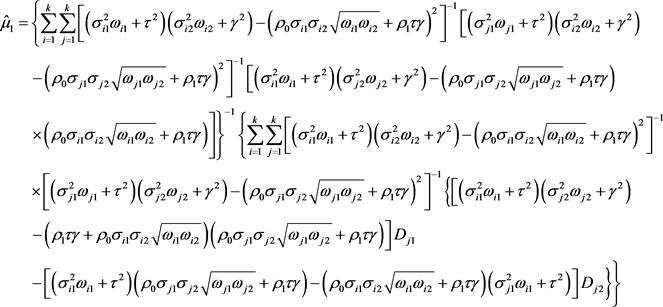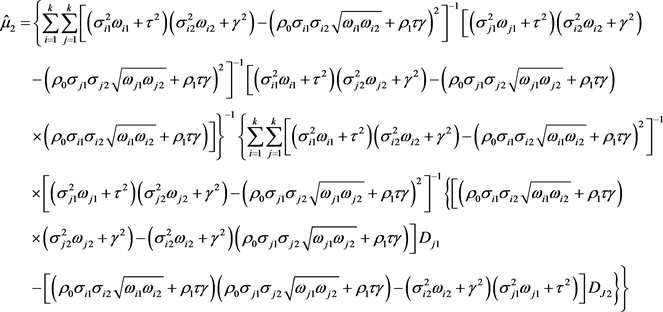${\stackrel{^}{\mu }}_{ML}={\left[\underset{i=1}{\overset{k}{\sum }}{\left({S}_{i}+\Sigma \right)}^{-1}\right]}^{-1}\underset{i=1}{\overset{k}{\sum }}{\left({S}_{i}+\Sigma \right)}^{-1}{D}_{i}$$\begin{array}{l}=\left\{\underset{i=1}{\overset{k}{\sum }}\underset{j=1}{\overset{k}{\sum }}{\left[\left({\sigma }_{i1}^{2}{\omega }_{i1}+{\tau }^{2}\right)\left({\sigma }_{i2}^{2}{\omega }_{i2}+{\gamma }^{2}\right)-{\left({\rho }_{0}{\sigma }_{i1}{\sigma }_{i2}\sqrt{{\omega }_{i1}{\omega }_{i2}}+{\rho }_{1}\tau \gamma \right)}^{2}\right]}^{-1}\left[\left({\sigma }_{j1}^{2}{\omega }_{j1}+{\tau }^{2}\right)\left({\sigma }_{i2}^{2}{\omega }_{i2}+{\gamma }^{2}\right)\\ \text{\hspace{0.17em}}\text{\hspace{0.17em}}-{{\left({\rho }_{0}{\sigma }_{j1}{\sigma }_{j2}\sqrt{{\omega }_{j1}{\omega }_{j2}}+{\rho }_{1}\tau \gamma \right)}^{2}\right]}^{-1}\left[\left({\sigma }_{i1}^{2}{\omega }_{i1}+{\tau }^{2}\right)\left({\sigma }_{j2}^{2}{\omega }_{j2}+{\gamma }^{2}\right)-\left({\rho }_{0}{\sigma }_{j1}{\sigma }_{j2}\sqrt{{\omega }_{j1}{\omega }_{j2}}+{\rho }_{1}\tau \gamma \right)\\ {\begin{array}{c}\text{ }\\ \text{ }\end{array}×\left({\rho }_{0}{\sigma }_{i1}{\sigma }_{i2}\sqrt{{\omega }_{i1}{\omega }_{i2}}+{\rho }_{1}\tau \gamma \right)\right]\right\}}^{-1}\left\{\underset{i=1}{\overset{k}{\sum }}\underset{j=1}{\overset{k}{\sum }}{\left[\left({\sigma }_{i1}^{2}{\omega }_{i1}+{\tau }^{2}\right)\left({\sigma }_{i2}^{2}{\omega }_{i2}+{\gamma }^{2}\right)-{\left({\rho }_{0}{\sigma }_{i1}{\sigma }_{i2}\sqrt{{\omega }_{i1}{\omega }_{i2}}+{\rho }_{1}\tau \gamma \right)}^{2}\right]}^{-1}\\ \text{\hspace{0.17em}}\text{\hspace{0.17em}}×{\left[\left({\sigma }_{j1}^{2}{\omega }_{j1}+{\tau }^{2}\right)\left({\sigma }_{j2}^{2}{\omega }_{j2}+{\gamma }^{2}\right)-{\left({\rho }_{0}{\sigma }_{j1}{\sigma }_{j2}\sqrt{{\omega }_{j1}{\omega }_{j2}}+{\rho }_{1}\tau \gamma \right)}^{2}\right]}^{-1}\left\{\left[\left({\sigma }_{i1}^{2}{\omega }_{i1}+{\tau }^{2}\right)\left({\sigma }_{j2}^{2}{\omega }_{j2}+{\gamma }^{2}\right)\\ \text{\hspace{0.17em}}\text{\hspace{0.17em}}-\left({\rho }_{1}\tau \gamma +{\rho }_{0}{\sigma }_{i1}{\sigma }_{i2}\sqrt{{\omega }_{i1}{\omega }_{i2}}\right)\left({\rho }_{0}{\sigma }_{j1}{\sigma }_{j2}\sqrt{{\omega }_{j1}{\omega }_{j2}}+{\rho }_{1}\tau \gamma \right)\right]E\left({D}_{j1}\right)\\ \text{\hspace{0.17em}}\begin{array}{c}\text{ }\\ \text{ }\end{array}-\left[\left({\sigma }_{i1}^{2}{\omega }_{i1}+{\tau }^{2}\right)\left({\rho }_{0}{\sigma }_{j1}{\sigma }_{j2}\sqrt{{\omega }_{j1}{\omega }_{j2}}+{\rho }_{1}\tau \gamma \right)-\left({\rho }_{0}{\sigma }_{i1}{\sigma }_{i2}\sqrt{{\omega }_{i1}{\omega }_{i2}}+{\rho }_{1}\tau \gamma \right)\left({\sigma }_{j1}^{2}{\omega }_{i1}+{\tau }^{2}\right)\right]E\left({D}_{j2}\right)\right\}\right\}\end{array}$

$\begin{array}{l}=\left\{\underset{i=1}{\overset{k}{\sum }}\underset{j=1}{\overset{k}{\sum }}{\left[\left({\sigma }_{i1}^{2}{\omega }_{i1}+{\tau }^{2}\right)\left({\sigma }_{i2}^{2}{\omega }_{i2}+{\gamma }^{2}\right)-{\left({\rho }_{0}{\sigma }_{i1}{\sigma }_{i2}\sqrt{{\omega }_{i1}{\omega }_{i2}}+{\rho }_{1}\tau \gamma \right)}^{2}\right]}^{-1}\left[\left({\sigma }_{j1}^{2}{\omega }_{j1}+{\tau }^{2}\right)\left({\sigma }_{i2}^{2}{\omega }_{i2}+{\gamma }^{2}\right)\\ \text{\hspace{0.17em}}\text{\hspace{0.17em}}-{{\left({\rho }_{0}{\sigma }_{j1}{\sigma }_{j2}\sqrt{{\omega }_{j1}{\omega }_{j2}}+{\rho }_{1}\tau \gamma \right)}^{2}\right]}^{-1}\left[\left({\sigma }_{i1}^{2}{\omega }_{i1}+{\tau }^{2}\right)\left({\sigma }_{j2}^{2}{\omega }_{j2}+{\gamma }^{2}\right)-\left({\rho }_{0}{\sigma }_{j1}{\sigma }_{j2}\sqrt{{\omega }_{j1}{\omega }_{j2}}+{\rho }_{1}\tau \gamma \right)\\ {\begin{array}{c}\text{ }\\ \text{ }\end{array}×\left({\rho }_{0}{\sigma }_{i1}{\sigma }_{i2}\sqrt{{\omega }_{i1}{\omega }_{i2}}+{\rho }_{1}\tau \gamma \right)\right]\right\}}^{-1}\left\{\underset{i=1}{\overset{k}{\sum }}\underset{j=1}{\overset{k}{\sum }}{\left[\left({\sigma }_{i1}^{2}{\omega }_{i1}+{\tau }^{2}\right)\left({\sigma }_{i2}^{2}{\omega }_{i2}+{\gamma }^{2}\right)-{\left({\rho }_{0}{\sigma }_{i1}{\sigma }_{i2}\sqrt{{\omega }_{i1}{\omega }_{i2}}+{\rho }_{1}\tau \gamma \right)}^{2}\right]}^{-1}\\ \text{\hspace{0.17em}}\text{\hspace{0.17em}}×{\left[\left({\sigma }_{j1}^{2}{\omega }_{j1}+{\tau }^{2}\right)\left({\sigma }_{j2}^{2}{\omega }_{j2}+{\gamma }^{2}\right)-{\left({\rho }_{0}{\sigma }_{j1}{\sigma }_{j2}\sqrt{{\omega }_{j1}{\omega }_{j2}}+{\rho }_{1}\tau \gamma \right)}^{2}\right]}^{-1}\left\{\left[\left({\sigma }_{i1}^{2}{\omega }_{i1}+{\tau }^{2}\right)\left({\sigma }_{j2}^{2}{\omega }_{j2}+{\gamma }^{2}\right)\\ \text{\hspace{0.17em}}\text{\hspace{0.17em}}-\left({\rho }_{1}\tau \gamma +{\rho }_{0}{\sigma }_{i1}{\sigma }_{i2}\sqrt{{\omega }_{i1}{\omega }_{i2}}\right)\left({\rho }_{0}{\sigma }_{j1}{\sigma }_{j2}\sqrt{{\omega }_{j1}{\omega }_{j2}}+{\rho }_{1}\tau \gamma \right)\right]{\mu }_{1}\\ \text{\hspace{0.17em}}\begin{array}{c}\text{ }\\ \text{ }\end{array}-\left[\left({\sigma }_{i1}^{2}{\omega }_{i1}+{\tau }^{2}\right)\left({\rho }_{0}{\sigma }_{j1}{\sigma }_{j2}\sqrt{{\omega }_{j1}{\omega }_{j2}}+{\rho }_{1}\tau \gamma \right)-\left({\rho }_{0}{\sigma }_{i1}{\sigma }_{i2}\sqrt{{\omega }_{i1}{\omega }_{i2}}+{\rho }_{1}\tau \gamma \right)\left({\sigma }_{j1}^{2}{\omega }_{i1}+{\tau }^{2}\right)\right]{\mu }_{2}\right\}\right\}\end{array}$

$\begin{array}{l}=\left\{\underset{i=1}{\overset{k}{\sum }}\underset{j=1}{\overset{k}{\sum }}{\left[\left({\sigma }_{i1}^{2}{\omega }_{i1}+{\tau }^{2}\right)\left({\sigma }_{i2}^{2}{\omega }_{i2}+{\gamma }^{2}\right)-{\left({\rho }_{0}{\sigma }_{i1}{\sigma }_{i2}\sqrt{{\omega }_{i1}{\omega }_{i2}}+{\rho }_{1}\tau \gamma \right)}^{2}\right]}^{-1}\left[\left({\sigma }_{j1}^{2}{\omega }_{j1}+{\tau }^{2}\right)\left({\sigma }_{i2}^{2}{\omega }_{i2}+{\gamma }^{2}\right)\\ \text{\hspace{0.17em}}\text{\hspace{0.17em}}-{{\left({\rho }_{0}{\sigma }_{j1}{\sigma }_{j2}\sqrt{{\omega }_{j1}{\omega }_{j2}}+{\rho }_{1}\tau \gamma \right)}^{2}\right]}^{-1}\left[\left({\sigma }_{i1}^{2}{\omega }_{i1}+{\tau }^{2}\right)\left({\sigma }_{j2}^{2}{\omega }_{j2}+{\gamma }^{2}\right)-\left({\rho }_{0}{\sigma }_{j1}{\sigma }_{j2}\sqrt{{\omega }_{j1}{\omega }_{j2}}+{\rho }_{1}\tau \gamma \right)\\ {\begin{array}{c}\text{ }\\ \text{ }\end{array}×\left({\rho }_{0}{\sigma }_{i1}{\sigma }_{i2}\sqrt{{\omega }_{i1}{\omega }_{i2}}+{\rho }_{1}\tau \gamma \right)\right]\right\}}^{-1}\left\{\underset{i=1}{\overset{k}{\sum }}\underset{j=1}{\overset{k}{\sum }}{\left[\left({\sigma }_{i1}^{2}{\omega }_{i1}+{\tau }^{2}\right)\left({\sigma }_{i2}^{2}{\omega }_{i2}+{\gamma }^{2}\right)-{\left({\rho }_{0}{\sigma }_{i1}{\sigma }_{i2}\sqrt{{\omega }_{i1}{\omega }_{i2}}+{\rho }_{1}\tau \gamma \right)}^{2}\right]}^{-1}\\ \text{\hspace{0.17em}}\text{\hspace{0.17em}}×{\left[\left({\sigma }_{j1}^{2}{\omega }_{j1}+{\tau }^{2}\right)\left({\sigma }_{j2}^{2}{\omega }_{j2}+{\gamma }^{2}\right)-{\left({\rho }_{0}{\sigma }_{j1}{\sigma }_{j2}\sqrt{{\omega }_{j1}{\omega }_{j2}}+{\rho }_{1}\tau \gamma \right)}^{2}\right]}^{-1}\left\{\left[\left({\sigma }_{i1}^{2}{\omega }_{i1}+{\tau }^{2}\right)\left({\sigma }_{j2}^{2}{\omega }_{j2}+{\gamma }^{2}\right)\\ \text{\hspace{0.17em}}\text{\hspace{0.17em}}-\left({\rho }_{1}\tau \gamma +{\rho }_{0}{\sigma }_{i1}{\sigma }_{i2}\sqrt{{\omega }_{i1}{\omega }_{i2}}\right)\left({\rho }_{0}{\sigma }_{j1}{\sigma }_{j2}\sqrt{{\omega }_{j1}{\omega }_{j2}}+{\rho }_{1}\tau \gamma \right)\right]{\mu }_{1}\\ \text{\hspace{0.17em}}\begin{array}{c}\text{ }\\ \text{ }\end{array}-\left[\left({\sigma }_{i1}^{2}{\omega }_{i1}+{\tau }^{2}\right)\left({\rho }_{0}{\sigma }_{j1}{\sigma }_{j2}\sqrt{{\omega }_{j1}{\omega }_{j2}}+{\rho }_{1}\tau \gamma \right)-\left({\rho }_{0}{\sigma }_{j1}{\sigma }_{j2}\sqrt{{\omega }_{j1}{\omega }_{j2}}+{\rho }_{1}\tau \gamma \right)\left({\sigma }_{i1}^{2}{\omega }_{i1}+{\tau }^{2}\right)\right]{\mu }_{2}\right\}\right\}\end{array}$

$\begin{array}{l}=\left\{\underset{i=1}{\overset{k}{\sum }}\underset{j=1}{\overset{k}{\sum }}{\left[\left({\sigma }_{i1}^{2}{\omega }_{i1}+{\tau }^{2}\right)\left({\sigma }_{i2}^{2}{\omega }_{i2}+{\gamma }^{2}\right)-{\left({\rho }_{0}{\sigma }_{i1}{\sigma }_{i2}\sqrt{{\omega }_{i1}{\omega }_{i2}}+{\rho }_{1}\tau \gamma \right)}^{2}\right]}^{-1}\left[\left({\sigma }_{j1}^{2}{\omega }_{j1}+{\tau }^{2}\right)\left({\sigma }_{i2}^{2}{\omega }_{i2}+{\gamma }^{2}\right)\\ \text{\hspace{0.17em}}\text{\hspace{0.17em}}-{{\left({\rho }_{0}{\sigma }_{j1}{\sigma }_{j2}\sqrt{{\omega }_{j1}{\omega }_{j2}}+{\rho }_{1}\tau \gamma \right)}^{2}\right]}^{-1}\left[\left({\sigma }_{i1}^{2}{\omega }_{i1}+{\tau }^{2}\right)\left({\sigma }_{j2}^{2}{\omega }_{j2}+{\gamma }^{2}\right)-\left({\rho }_{0}{\sigma }_{j1}{\sigma }_{j2}\sqrt{{\omega }_{j1}{\omega }_{j2}}+{\rho }_{1}\tau \gamma \right)\\ {\begin{array}{c}\text{ }\\ \text{ }\end{array}×\left({\rho }_{0}{\sigma }_{i1}{\sigma }_{i2}\sqrt{{\omega }_{i1}{\omega }_{i2}}+{\rho }_{1}\tau \gamma \right)\right]\right\}}^{-1}\left\{\underset{i=1}{\overset{k}{\sum }}\underset{j=1}{\overset{k}{\sum }}{\left[\left({\sigma }_{i1}^{2}{\omega }_{i1}+{\tau }^{2}\right)\left({\sigma }_{i2}^{2}{\omega }_{i2}+{\gamma }^{2}\right)-{\left({\rho }_{0}{\sigma }_{i1}{\sigma }_{i2}\sqrt{{\omega }_{i1}{\omega }_{i2}}+{\rho }_{1}\tau \gamma \right)}^{2}\right]}^{-1}\\ \text{\hspace{0.17em}}\text{\hspace{0.17em}}×{\left[\left({\sigma }_{j1}^{2}{\omega }_{j1}+{\tau }^{2}\right)\left({\sigma }_{j2}^{2}{\omega }_{j2}+{\gamma }^{2}\right)-{\left({\rho }_{0}{\sigma }_{j1}{\sigma }_{j2}\sqrt{{\omega }_{j1}{\omega }_{j2}}+{\rho }_{1}\tau \gamma \right)}^{2}\right]}^{-1}\left[\left({\sigma }_{i1}^{2}{\omega }_{i1}+{\tau }^{2}\right)\left({\sigma }_{j2}^{2}{\omega }_{j2}+{\gamma }^{2}\right)\\ \text{\hspace{0.17em}}\begin{array}{c}\text{ }\\ \text{ }\end{array}-\left({\rho }_{1}\tau \gamma +{\rho }_{0}{\sigma }_{i1}{\sigma }_{i2}\sqrt{{\omega }_{i1}{\omega }_{i2}}\right)\left({\rho }_{0}{\sigma }_{j1}{\sigma }_{j2}\sqrt{{\omega }_{j1}{\omega }_{j2}}+{\rho }_{1}\tau \gamma \right)\right]\right\}{\mu }_{1}\\ ={\mu }_{1}\end{array}$$\begin{array}{l}=\left\{\underset{i=1}{\overset{k}{\sum }}\underset{j=1}{\overset{k}{\sum }}{\left[\left({\sigma }_{i1}^{2}{\omega }_{i1}+{\tau }^{2}\right)\left({\sigma }_{i2}^{2}{\omega }_{i2}+{\gamma }^{2}\right)-{\left({\rho }_{0}{\sigma }_{i1}{\sigma }_{i2}\sqrt{{\omega }_{i1}{\omega }_{i2}}+{\rho }_{1}\tau \gamma \right)}^{2}\right]}^{-1}\left[\left({\sigma }_{j1}^{2}{\omega }_{j1}+{\tau }^{2}\right)\left({\sigma }_{i2}^{2}{\omega }_{i2}+{\gamma }^{2}\right)\\ \text{\hspace{0.17em}}\text{\hspace{0.17em}}-{{\left({\rho }_{0}{\sigma }_{j1}{\sigma }_{j2}\sqrt{{\omega }_{j1}{\omega }_{j2}}+{\rho }_{1}\tau \gamma \right)}^{2}\right]}^{-1}\left[\left({\sigma }_{i1}^{2}{\omega }_{i1}+{\tau }^{2}\right)\left({\sigma }_{j2}^{2}{\omega }_{j2}+{\gamma }^{2}\right)-\left({\rho }_{0}{\sigma }_{j1}{\sigma }_{j2}\sqrt{{\omega }_{j1}{\omega }_{j2}}+{\rho }_{1}\tau \gamma \right)\\ \text{\hspace{0.17em}}{\begin{array}{c}\text{ }\\ \text{ }\end{array}×\left({\rho }_{0}{\sigma }_{i1}{\sigma }_{i2}\sqrt{{\omega }_{i1}{\omega }_{i2}}+{\rho }_{1}\tau \gamma \right)\right]\right\}}^{-1}\left\{\underset{i=1}{\overset{k}{\sum }}\underset{j=1}{\overset{k}{\sum }}{\left[\left({\sigma }_{i1}^{2}{\omega }_{i1}+{\tau }^{2}\right)\left({\sigma }_{i2}^{2}{\omega }_{i2}+{\gamma }^{2}\right)-{\left({\rho }_{0}{\sigma }_{i1}{\sigma }_{i2}\sqrt{{\omega }_{i1}{\omega }_{i2}}+{\rho }_{1}\tau \gamma \right)}^{2}\right]}^{-1}\\ \text{\hspace{0.17em}}\text{\hspace{0.17em}}×{\left[\left({\sigma }_{j1}^{2}{\omega }_{j1}+{\tau }^{2}\right)\left({\sigma }_{j2}^{2}{\omega }_{j2}+{\gamma }^{2}\right)-{\left({\rho }_{0}{\sigma }_{j1}{\sigma }_{j2}\sqrt{{\omega }_{j1}{\omega }_{j2}}+{\rho }_{1}\tau \gamma \right)}^{2}\right]}^{-1}\left\{\left[\left({\rho }_{0}{\sigma }_{i1}{\sigma }_{i2}\sqrt{{\omega }_{i1}{\omega }_{i2}}+{\rho }_{1}\tau \gamma \right)\\ \text{\hspace{0.17em}}\text{\hspace{0.17em}}×\left({\sigma }_{j2}^{2}{\omega }_{j2}+{\gamma }^{2}\right)-\left({\sigma }_{i2}^{2}{\omega }_{i2}+{\gamma }^{2}\right)\left({\rho }_{0}{\sigma }_{j1}{\sigma }_{j2}\sqrt{{\omega }_{j1}{\omega }_{j2}}+{\rho }_{1}\tau \gamma \right)\right]E\left({D}_{j1}\right)\\ \text{\hspace{0.17em}}\begin{array}{c}\text{ }\\ \text{ }\end{array}-\left[\left({\rho }_{1}\tau \gamma +{\rho }_{0}{\sigma }_{i1}{\sigma }_{i2}\sqrt{{\omega }_{i1}{\omega }_{i2}}\right)\left({\rho }_{0}{\sigma }_{j1}{\sigma }_{j2}\sqrt{{\omega }_{j1}{\omega }_{j2}}+{\rho }_{1}\tau \gamma \right)-\left({\sigma }_{i2}^{2}{\omega }_{i2}+{\gamma }^{2}\right)\left({\sigma }_{j1}^{2}{\omega }_{j1}+{\tau }^{2}\right)\right]E\left({D}_{J2}\right)\right\}\right\}\end{array}$

$\begin{array}{l}=\left\{\underset{i=1}{\overset{k}{\sum }}\underset{j=1}{\overset{k}{\sum }}{\left[\left({\sigma }_{i1}^{2}{\omega }_{i1}+{\tau }^{2}\right)\left({\sigma }_{i2}^{2}{\omega }_{i2}+{\gamma }^{2}\right)-{\left({\rho }_{0}{\sigma }_{i1}{\sigma }_{i2}\sqrt{{\omega }_{i1}{\omega }_{i2}}+{\rho }_{1}\tau \gamma \right)}^{2}\right]}^{-1}\left[\left({\sigma }_{j1}^{2}{\omega }_{j1}+{\tau }^{2}\right)\left({\sigma }_{i2}^{2}{\omega }_{i2}+{\gamma }^{2}\right)\\ \text{\hspace{0.17em}}\text{\hspace{0.17em}}-{{\left({\rho }_{0}{\sigma }_{j1}{\sigma }_{j2}\sqrt{{\omega }_{j1}{\omega }_{j2}}+{\rho }_{1}\tau \gamma \right)}^{2}\right]}^{-1}\left[\left({\sigma }_{i1}^{2}{\omega }_{i1}+{\tau }^{2}\right)\left({\sigma }_{j2}^{2}{\omega }_{j2}+{\gamma }^{2}\right)-\left({\rho }_{0}{\sigma }_{j1}{\sigma }_{j2}\sqrt{{\omega }_{j1}{\omega }_{j2}}+{\rho }_{1}\tau \gamma \right)\\ \text{\hspace{0.17em}}{\begin{array}{c}\text{ }\\ \text{ }\end{array}×\left({\rho }_{0}{\sigma }_{i1}{\sigma }_{i2}\sqrt{{\omega }_{i1}{\omega }_{i2}}+{\rho }_{1}\tau \gamma \right)\right]\right\}}^{-1}\left\{\underset{i=1}{\overset{k}{\sum }}\underset{j=1}{\overset{k}{\sum }}{\left[\left({\sigma }_{i1}^{2}{\omega }_{i1}+{\tau }^{2}\right)\left({\sigma }_{i2}^{2}{\omega }_{i2}+{\gamma }^{2}\right)-{\left({\rho }_{0}{\sigma }_{i1}{\sigma }_{i2}\sqrt{{\omega }_{i1}{\omega }_{i2}}+{\rho }_{1}\tau \gamma \right)}^{2}\right]}^{-1}\\ \text{\hspace{0.17em}}\text{\hspace{0.17em}}×{\left[\left({\sigma }_{j1}^{2}{\omega }_{j1}+{\tau }^{2}\right)\left({\sigma }_{j2}^{2}{\omega }_{j2}+{\gamma }^{2}\right)-{\left({\rho }_{0}{\sigma }_{j1}{\sigma }_{j2}\sqrt{{\omega }_{j1}{\omega }_{j2}}+{\rho }_{1}\tau \gamma \right)}^{2}\right]}^{-1}\left\{\left[\left({\rho }_{0}{\sigma }_{i1}{\sigma }_{i2}\sqrt{{\omega }_{i1}{\omega }_{i2}}+{\rho }_{1}\tau \gamma \right)\\ \text{\hspace{0.17em}}\text{\hspace{0.17em}}×\left({\sigma }_{j2}^{2}{\omega }_{j2}+{\gamma }^{2}\right)-\left({\sigma }_{i2}^{2}{\omega }_{i2}+{\gamma }^{2}\right)\left({\rho }_{0}{\sigma }_{j1}{\sigma }_{j2}\sqrt{{\omega }_{j1}{\omega }_{j2}}+{\rho }_{1}\tau \gamma \right)\right]{\mu }_{1}\\ \text{\hspace{0.17em}}\begin{array}{c}\text{ }\\ \text{ }\end{array}-\left[\left({\rho }_{1}\tau \gamma +{\rho }_{0}{\sigma }_{i1}{\sigma }_{i2}\sqrt{{\omega }_{i1}{\omega }_{i2}}\right)\left({\rho }_{0}{\sigma }_{j1}{\sigma }_{j2}\sqrt{{\omega }_{j1}{\omega }_{j2}}+{\rho }_{1}\tau \gamma \right)-\left({\sigma }_{i2}^{2}{\omega }_{i2}+{\gamma }^{2}\right)\left({\sigma }_{j1}^{2}{\omega }_{j1}+{\tau }^{2}\right)\right]{\mu }_{2}\right\}\right\}\end{array}$

$\begin{array}{l}=\left\{\underset{i=1}{\overset{k}{\sum }}\underset{j=1}{\overset{k}{\sum }}{\left[\left({\sigma }_{i1}^{2}{\omega }_{i1}+{\tau }^{2}\right)\left({\sigma }_{i2}^{2}{\omega }_{i2}+{\gamma }^{2}\right)-{\left({\rho }_{0}{\sigma }_{i1}{\sigma }_{i2}\sqrt{{\omega }_{i1}{\omega }_{i2}}+{\rho }_{1}\tau \gamma \right)}^{2}\right]}^{-1}\left[\left({\sigma }_{j1}^{2}{\omega }_{j1}+{\tau }^{2}\right)\left({\sigma }_{i2}^{2}{\omega }_{i2}+{\gamma }^{2}\right)\\ \text{\hspace{0.17em}}\text{\hspace{0.17em}}-{{\left({\rho }_{0}{\sigma }_{j1}{\sigma }_{j2}\sqrt{{\omega }_{j1}{\omega }_{j2}}+{\rho }_{1}\tau \gamma \right)}^{2}\right]}^{-1}\left[\left({\sigma }_{i1}^{2}{\omega }_{i1}+{\tau }^{2}\right)\left({\sigma }_{j2}^{2}{\omega }_{j2}+{\gamma }^{2}\right)-\left({\rho }_{0}{\sigma }_{j1}{\sigma }_{j2}\sqrt{{\omega }_{j1}{\omega }_{j2}}+{\rho }_{1}\tau \gamma \right)\\ \text{\hspace{0.17em}}{\begin{array}{c}\text{ }\\ \text{ }\end{array}×\left({\rho }_{0}{\sigma }_{i1}{\sigma }_{i2}\sqrt{{\omega }_{i1}{\omega }_{i2}}+{\rho }_{1}\tau \gamma \right)\right]\right\}}^{-1}\left\{\underset{i=1}{\overset{k}{\sum }}\underset{j=1}{\overset{k}{\sum }}{\left[\left({\sigma }_{i1}^{2}{\omega }_{i1}+{\tau }^{2}\right)\left({\sigma }_{i2}^{2}{\omega }_{i2}+{\gamma }^{2}\right)-{\left({\rho }_{0}{\sigma }_{i1}{\sigma }_{i2}\sqrt{{\omega }_{i1}{\omega }_{i2}}+{\rho }_{1}\tau \gamma \right)}^{2}\right]}^{-1}\\ \text{\hspace{0.17em}}\text{\hspace{0.17em}}×{\left[\left({\sigma }_{j1}^{2}{\omega }_{j1}+{\tau }^{2}\right)\left({\sigma }_{j2}^{2}{\omega }_{j2}+{\gamma }^{2}\right)-{\left({\rho }_{0}{\sigma }_{j1}{\sigma }_{j2}\sqrt{{\omega }_{j1}{\omega }_{j2}}+{\rho }_{1}\tau \gamma \right)}^{2}\right]}^{-1}\left\{\left[\left({\rho }_{0}{\sigma }_{i1}{\sigma }_{i2}\sqrt{{\omega }_{i1}{\omega }_{i2}}+{\rho }_{1}\tau \gamma \right)\\ \text{\hspace{0.17em}}\text{\hspace{0.17em}}×\left({\sigma }_{j2}^{2}{\omega }_{j2}+{\gamma }^{2}\right)-\left({\sigma }_{j2}^{2}{\omega }_{j2}+{\gamma }^{2}\right)\left({\rho }_{0}{\sigma }_{i1}{\sigma }_{i2}\sqrt{{\omega }_{i1}{\omega }_{i2}}+{\rho }_{1}\tau \gamma \right)\right]{\mu }_{1}\\ \text{\hspace{0.17em}}\begin{array}{c}\text{ }\\ \text{ }\end{array}-\left[\left({\rho }_{1}\tau \gamma +{\rho }_{0}{\sigma }_{i1}{\sigma }_{i2}\sqrt{{\omega }_{i1}{\omega }_{i2}}\right)\left({\rho }_{0}{\sigma }_{j1}{\sigma }_{j2}\sqrt{{\omega }_{j1}{\omega }_{j2}}+{\rho }_{1}\tau \gamma \right)-\left({\sigma }_{i2}^{2}{\omega }_{i2}+{\gamma }^{2}\right)\left({\sigma }_{j1}^{2}{\omega }_{j1}+{\tau }^{2}\right)\right]{\mu }_{2}\right\}\right\}\end{array}$

$\begin{array}{l}=\left\{\underset{i=1}{\overset{k}{\sum }}\underset{j=1}{\overset{k}{\sum }}{\left[\left({\sigma }_{i1}^{2}{\omega }_{i1}+{\tau }^{2}\right)\left({\sigma }_{i2}^{2}{\omega }_{i2}+{\gamma }^{2}\right)-{\left({\rho }_{0}{\sigma }_{i1}{\sigma }_{i2}\sqrt{{\omega }_{i1}{\omega }_{i2}}+{\rho }_{1}\tau \gamma \right)}^{2}\right]}^{-1}\left[\left({\sigma }_{j1}^{2}{\omega }_{j1}+{\tau }^{2}\right)\left({\sigma }_{i2}^{2}{\omega }_{i2}+{\gamma }^{2}\right)\\ \text{\hspace{0.17em}}\text{\hspace{0.17em}}-{{\left({\rho }_{0}{\sigma }_{j1}{\sigma }_{j2}\sqrt{{\omega }_{j1}{\omega }_{j2}}+{\rho }_{1}\tau \gamma \right)}^{2}\right]}^{-1}\left[\left({\sigma }_{i1}^{2}{\omega }_{i1}+{\tau }^{2}\right)\left({\sigma }_{j2}^{2}{\omega }_{j2}+{\gamma }^{2}\right)-\left({\rho }_{0}{\sigma }_{j1}{\sigma }_{j2}\sqrt{{\omega }_{j1}{\omega }_{j2}}+{\rho }_{1}\tau \gamma \right)\\ \text{\hspace{0.17em}}{\begin{array}{c}\text{ }\\ \text{ }\end{array}×\left({\rho }_{0}{\sigma }_{i1}{\sigma }_{i2}\sqrt{{\omega }_{i1}{\omega }_{i2}}+{\rho }_{1}\tau \gamma \right)\right]\right\}}^{-1}\left\{\underset{i=1}{\overset{k}{\sum }}\underset{j=1}{\overset{k}{\sum }}{\left[\left({\sigma }_{i1}^{2}{\omega }_{i1}+{\tau }^{2}\right)\left({\sigma }_{i2}^{2}{\omega }_{i2}+{\gamma }^{2}\right)-{\left({\rho }_{0}{\sigma }_{i1}{\sigma }_{i2}\sqrt{{\omega }_{i1}{\omega }_{i2}}+{\rho }_{1}\tau \gamma \right)}^{2}\right]}^{-1}\\ \text{\hspace{0.17em}}\text{\hspace{0.17em}}×{\left[\left({\sigma }_{j1}^{2}{\omega }_{j1}+{\tau }^{2}\right)\left({\sigma }_{j2}^{2}{\omega }_{j2}+{\gamma }^{2}\right)-{\left({\rho }_{0}{\sigma }_{j1}{\sigma }_{j2}\sqrt{{\omega }_{j1}{\omega }_{j2}}+{\rho }_{1}\tau \gamma \right)}^{2}\right]}^{-1}\\ \text{\hspace{0.17em}}\begin{array}{c}\text{ }\\ \text{ }\end{array}×\left[\left({\sigma }_{j2}^{2}{\omega }_{j2}+{\gamma }^{2}\right)\left({\sigma }_{i1}^{2}{\omega }_{i1}+{\tau }^{2}\right)-\left({\rho }_{0}{\sigma }_{j1}{\sigma }_{j2}\sqrt{{\omega }_{j1}{\omega }_{j2}}+{\rho }_{1}\tau \gamma \right)\left({\rho }_{0}{\sigma }_{i1}{\sigma }_{i2}\sqrt{{\omega }_{i1}{\omega }_{i2}}+{\rho }_{1}\tau \gamma \right)\right]{\mu }_{2}\right\}\\ ={\mu }_{2}\end{array}$

4. 均值效应量极大似然估计量的协方差矩阵

$Var{\left({\stackrel{^}{\mu }}_{ML}\right)}_{\left(1,1\right)}={\left\{det\left[\underset{i=1}{\overset{k}{\sum }}{\left({S}_{i}+\Sigma \right)}^{-1}\right]\right\}}^{-1}\left\{\underset{i=1}{\overset{k}{\sum }}det{\left[\left({S}_{i}+\Sigma \right)\right]}^{-1}\left({\sigma }_{i1}^{2}{\omega }_{i1}+{\tau }^{2}\right)\right\}$

$Var{\left({\stackrel{^}{\mu }}_{ML}\right)}_{\left(1,2\right)}={\left\{det\left[\underset{i=1}{\overset{k}{\sum }}{\left({S}_{i}+\Sigma \right)}^{-1}\right]\right\}}^{-1}\left\{\underset{i=1}{\overset{k}{\sum }}det{\left[\left({S}_{i}+\Sigma \right)\right]}^{-1}\left({\rho }_{0}{\sigma }_{i1}{\sigma }_{i2}\sqrt{{\omega }_{i1}{\omega }_{i2}}+{\rho }_{1}\tau \gamma \right)\right\}$

$Var{\left({\stackrel{^}{\mu }}_{ML}\right)}_{\left(2,1\right)}={\left\{det\left[\underset{i=1}{\overset{k}{\sum }}{\left({S}_{i}+\Sigma \right)}^{-1}\right]\right\}}^{-1}\left\{\underset{i=1}{\overset{k}{\sum }}det{\left[\left({S}_{i}+\Sigma \right)\right]}^{-1}\left({\rho }_{0}{\sigma }_{i1}{\sigma }_{i2}\sqrt{{\omega }_{i1}{\omega }_{i2}}+{\rho }_{1}\tau \gamma \right)\right\}$

$Var{\left({\stackrel{^}{\mu }}_{ML}\right)}_{\left(2,2\right)}={\left\{det\left[\underset{i=1}{\overset{k}{\sum }}{\left({S}_{i}+\Sigma \right)}^{-1}\right]\right\}}^{-1}\left\{\underset{i=1}{\overset{k}{\sum }}det{\left[\left({S}_{i}+\Sigma \right)\right]}^{-1}\left({\sigma }_{i2}^{2}{\omega }_{i2}+{\gamma }^{2}\right)\right\}$

$\begin{array}{l}det\left[\underset{i=1}{\overset{k}{\sum }}{\left({S}_{i}+\Sigma \right)}^{-1}\right]\\ =\underset{i=1}{\overset{k}{\sum }}\underset{j=1}{\overset{k}{\sum }}{\left[\left({\sigma }_{i1}^{2}{\omega }_{i1}+{\tau }^{2}\right)\left({\sigma }_{i2}^{2}{\omega }_{i2}+{\gamma }^{2}\right)-{\left({\rho }_{0}{\sigma }_{i1}{\sigma }_{i2}\sqrt{{\omega }_{i1}{\omega }_{i2}}+{\rho }_{1}\tau \gamma \right)}^{2}\right]}^{-1}\\ \text{\hspace{0.17em}}\text{\hspace{0.17em}}×{\left[\left({\sigma }_{j1}^{2}{\omega }_{j1}+{\tau }^{2}\right)\left({\sigma }_{j2}^{2}{\omega }_{j2}+{\gamma }^{2}\right)-{\left({\rho }_{0}{\sigma }_{j1}{\sigma }_{j2}\sqrt{{\omega }_{j1}{\omega }_{j2}}+{\rho }_{1}\tau \gamma \right)}^{2}\right]}^{-1}\\ \text{\hspace{0.17em}}\text{\hspace{0.17em}}×\left[\left({\sigma }_{i1}^{2}{\omega }_{i1}+{\tau }^{2}\right)\left({\sigma }_{j2}^{2}{\omega }_{j2}+{\gamma }^{2}\right)-\left({\rho }_{0}{\sigma }_{j1}{\sigma }_{j2}\sqrt{{\omega }_{j1}{\omega }_{j2}}+{\rho }_{1}\tau \gamma \right)\left({\rho }_{0}{\sigma }_{i1}{\sigma }_{i2}\sqrt{{\omega }_{i1}{\omega }_{i2}}+{\rho }_{1}\tau \gamma \right)\right]\end{array}$

$det\left[{\left({S}_{i}+\Sigma \right)}^{-1}\right]=\underset{j=1}{\overset{k}{\sum }}\left[\left({\sigma }_{i1}^{2}{\omega }_{i1}+{\tau }^{2}\right)\left({\sigma }_{i2}^{2}{\omega }_{i2}+{\gamma }^{2}\right)-{\left({\rho }_{0}{\sigma }_{i1}{\sigma }_{i2}\sqrt{{\omega }_{i1}{\omega }_{i2}}+{\rho }_{1}\tau \gamma \right)}^{2}\right]$

$\begin{array}{c}Var\left({\stackrel{^}{\mu }}_{ML}\right)=Var\left\{{\left[\underset{i=1}{\overset{k}{\sum }}{\left({S}_{i}+\Sigma \right)}^{-1}\right]}^{-1}\underset{i=1}{\overset{k}{\sum }}{\left({S}_{i}+\Sigma \right)}^{-1}{D}_{i}\right\}\\ =Var\left\{\underset{i=1}{\overset{k}{\sum }}\left\{{\left[\underset{i=1}{\overset{k}{\sum }}{\left({S}_{i}+\Sigma \right)}^{-1}\right]}^{-1}{\left({S}_{i}+\Sigma \right)}^{-1}{D}_{i}\right\}\right\}\\ =\underset{i=1}{\overset{k}{\sum }}Var\left\{{\left[\underset{i=1}{\overset{k}{\sum }}{\left({S}_{i}+\Sigma \right)}^{-1}\right]}^{-1}{\left({S}_{i}+\Sigma \right)}^{-1}{D}_{i}\right\}\\ =\underset{i=1}{\overset{k}{\sum }}{\left\{{\left[\underset{i=1}{\overset{k}{\sum }}{\left({S}_{i}+\Sigma \right)}^{-1}\right]}^{-1}{\left({S}_{i}+\Sigma \right)}^{-1}\left({S}_{i}+\Sigma \right){\left({S}_{i}+\Sigma \right)}^{-1}\right\}}^{\text{T}}{\left\{{\left[\underset{i=1}{\overset{k}{\sum }}{\left({S}_{i}+\Sigma \right)}^{-1}\right]}^{-1}\right\}}^{\text{T}}\end{array}$

$\begin{array}{l}=\underset{i=1}{\overset{k}{\sum }}\left\{{\left[\underset{i=1}{\overset{k}{\sum }}{\left({S}_{i}+\Sigma \right)}^{-1}\right]}^{-1}{\left({S}_{i}+\Sigma \right)}^{-1}\left({S}_{i}+\Sigma \right){\left({S}_{i}+\Sigma \right)}^{-1}{\left[\underset{i=1}{\overset{k}{\sum }}{\left({S}_{i}+\Sigma \right)}^{-1}\right]}^{-1}\right\}\\ =\underset{i=1}{\overset{k}{\sum }}\left\{{\left[\underset{i=1}{\overset{k}{\sum }}{\left({S}_{i}+\Sigma \right)}^{-1}\right]}^{-1}{\left({S}_{i}+\Sigma \right)}^{-1}{\left[\underset{i=1}{\overset{k}{\sum }}{\left({S}_{i}+\Sigma \right)}^{-1}\right]}^{-1}\right\}\\ ={\left[\underset{i=1}{\overset{k}{\sum }}{\left({S}_{i}+\Sigma \right)}^{-1}\right]}^{-1}\left[\underset{i=1}{\overset{k}{\sum }}{\left({S}_{i}+\Sigma \right)}^{-1}\right]{\left[\underset{i=1}{\overset{k}{\sum }}{\left({S}_{i}+\Sigma \right)}^{-1}\right]}^{-1}\\ ={\left[\underset{i=1}{\overset{k}{\sum }}{\left({S}_{i}+\Sigma \right)}^{-1}\right]}^{-1}\end{array}$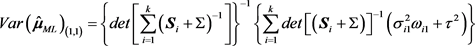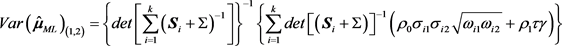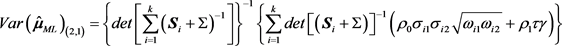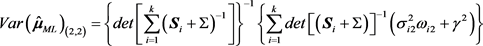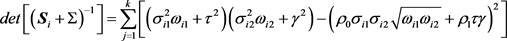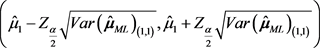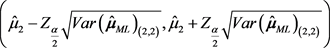，其中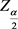表示正态分布的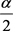分位数，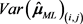表示矩阵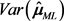第i行第j列的分量。

5. 结束语

The Random-Effect Model of Bivariate Meta-Analysis Based on the Difference Mean[J]. 理论数学, 2019, 09(01): 98-110. https://doi.org/10.12677/PM.2019.91013

1. 1. Pearson, K. (1904) Report on Certain Enteric Fever Inoculation Statistics. British Medical Journal, 3, 1243-1246.

2. 2. Chalmers, I., Hedges, L.V. and Cooper, H. (2002) A Brief History of Research Synthesis. Evaluation and the Health Professions, 25, 12-37.
https://doi.org/10.1177/0163278702025001003

3. 3. Lee, W.L., Bausell, R.B. and Berman, B.M. (2001) The Growth of Health-Related Meta-Analyses Published from 1980 to 2000. Evaluation and the Health Professions, 24, 327-335.
https://doi.org/10.1177/01632780122034948

4. 4. Normand, S.-L.T. (1998) Tutorial in Biostatistics Meta-Analysis: Formulating, Evaluating, Combining, and Reporting. Statistics in Medicine, 18, 321-359.

5. 5. Raudenbush, S.W., Becker, B.J. and Kalaian, H. (1988) Modeling Multivariate Effect Sizes. Psychological Bulletin, 103, 111-120.
https://doi.org/10.1037/0033-2909.103.1.111

6. 6. van Houwelingen, H.C., Zwinderman, K.H. and Stijnen, T. (1993) A Bivariate Approach to Meta-Analysis. Statistics in Medicine, 12, 2273-2284.
https://doi.org/10.1002/sim.4780122405

7. 7. Kalaian, H.A. and Raudenbush, S.W. (1996) A Multivariate Mixed Linear Model for Meta-Analysis. Psychological Methods, 1, 227-235.
https://doi.org/10.1037/1082-989X.1.3.227

8. 8. van Houwelingen, H.C., Arends, L.R. and Stijnen, T. (2002) Advanced Methods in Meta-Analysis: Multivariate Approach and Metaregression. Statistics in Medicine, 21, 589-624.
https://doi.org/10.1002/sim.1040

9. 9. Riley, R.D., Abrams, K.R., Lambert, P.C., Sutton, A.J. and Thompson, J.R. (2007) Bivariate Random Effects Meta-Analysis and the Estimation of Between-Study Correlation. BMC Medical Research Methodology, 7, 3.
https://doi.org/10.1186/1471-2288-7-3

10. 10. Ritz, J., Demidenko, E. and Spiegelman, D. (2008) Multivariate Meta-Analysis for Data Consortia, Individual Patient Meta-Analysis, and Pooling Projects. Journal of Statistical Plan-ning and Inference, 138, 1919-1933.
https://doi.org/10.1016/j.jspi.2007.07.004

11. 11. Paul, M., Riebler, A., Bachmann, L.M., Rue, H. and Held, L. (2010) Bayesian Bivariate Meta-Analysis of Diagnostic Test Studies Using Integrated Nested Laplace Approximations. Statistics in Medicine, 29, 1325-1339.
https://doi.org/10.1002/sim.3858

12. NOTES

*通讯作者。